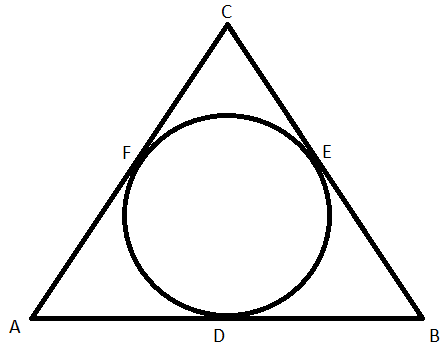QUESTION

# The semi perimeter of triangle ABC = 28 cm, then find the value of AF + BD + CE.(a) 28 cm(b) 16 cm(c) 12 cm(d) 19 cmHint: First, using the property of tangents from a point, find that AF = AD, DB = BE, and CF = CE. Then write the expression for the perimeter of triangle ABC as AB + BC + AC = 56 cm. Break AB, BC, and AC into (AD + BD), (BE + CE), and (AF + CF), respectively. Then, rearranging this and using the above found relations, we find the final answer.

In this question, we are given that the semi perimeter of triangle ABC = 28 cm.

We need to find the value of AF + BD + CE from the given figure.We can see from the figure that the sides of the triangle are tangents to the circle.
We know a property of tangents on a circle that the length of tangents from an external point to a circle are equal.

Using this property, we will get the following:

DB = BE

CF = CE

Now, we are given that the semi perimeter of the triangle ABC is 28 cm.

We know that the perimeter of a triangle is double of the semi perimeter. Using this property, we will get the following:

Perimeter of triangle ABC = 56 cm

AB + BC + AC = 56 cm

(AD + BD) + (BE + CE) + (AF + CF) = 56 cm

(AD + AF) + (BD + BE) + (CE + CF) = 56 cm

2(AF + BD +CE) = 56 cm

AF + BD + CE = 28 cm

So, option (a) is correct.

Note: In this question, it is very important to realise that the sides of the triangle are tangents to the circle. Also, it is very important to know the relation between the semi perimeter and the perimeter and the property that length of tangents from an external point to a circle are equal.